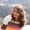Lessons: 20Length: 1.7 hours• Overview
• Transcript

# 2.5 Classes and Functions

In this lesson, I'll show you how to call other functions from Kotlin main(). You'll see how to call a function inside a class and how to use field variables and class properties outside the class.

## 5.Conclusion1 lesson, 01:17

### 2.5 Classes and Functions

Hello, in this lesson we will be learning about functions and classes in Kotlin. So let's get started without further delay. This is the main function that is executed at the very beginning when the app runs. Let us write a simple code here to define the variable and print it out. This is the plain, conventional code that we will write to define the variable, initialize it and print it out. Now let us do the same with the help of another function in Kotlin. Let us define another function, let's say display. We will have the argument here. Let's give it a name, figure, which will be of string type. Let us cut this line of code and write it down here. We have the parameter here as figure. So we will print the figure here. And now we will call the display function in which we will be passing element as the argument. So display element. We have called the display function, in which we are passing element as an argument. In the display function, we have the figure as the parameter. I have named it figure so that you can differentiate it easily from element. So the value of this element comes to the figure which is our string type. And this variable is printed here. Let me append a string here so that you can differentiate it easily. Element is figure. Now let us run the code and see what happens. Here we are. We have the output here as element is square. So we can derive the conclusion that this element is passed into this function and the variable is printed here. This is how we call one function from the other function. Now, let us deal with classes here. How we can call this function if it was inside a class. Let us define a class, say Geometry. And inside it, let us put this function. So here we have class Geometry in which we have the function display with the parameter as figure of string type. We can see the area here which says unresolved reference display. Since display is inside a class we need to define the class object using which we will call the display function inside it. So let us first define an object of Geometry class. Let's say objGeometry var objGeometry is equal to Geometry. Mark the difference here. If it were Java, we would have used a new keyword to create an object for the class, but in Kotlin, there is less ceremony. We don't need this new keyword. We only have to create the object by giving it the class name. Now using this object of Geometry class, we will call the display function in the Geometry class. The syntax to call a function which is inside a class is using the class object Dot the function name. And here we have the parameter that we will be passing to the display function of the Geometry class. Now let us run our code and see what happens. But before that, let me modify this a little bit so that you can differentiate it. So let's run and see what happens. And here we have element is equal to square. So we have successfully called the display function which is inside the Geometry class from our main class. Now we know how to use the functions and classes in Kotlin from our main function. Let us dive a little deeper into it and let us declare a field variable in the Geometry class. Let's say we have the variable figure. Now it says the property must be initialized or should be abstract. So let us initialize it to a blank string here and remove this parameter from the display function of Geometry class. Now we have this figure which we will be using in the display function. Let us remove this element from here. And now, we will initialize the field variable figure of the Geometry class in our main function. How do we do that? Same way like we access the functions. Use the object of the class. Dot figure is equal to Element. And here we have initialized the field variable of the Geometry class to our desired variable. Running it. Figure element is square. So here we have successfully initialized our field variable of the Geometry class and used it in the function of the Geometry class. Now instead of calling the display function, which prints the field variable, we can directly print this field variable in our main function. How do we do that? Let's copy this line of code. Paste it here. Let's comment it out. And now we write this. So we will print Element is objGeometry.figure. So here we are. We see how we can print the field variable of another class from our main function. We assess it using the object of the class. So summing up the lesson, we learned about functions. We learned about classes. How do we define the field variable? How do we instantiate the object of the class? And how do we use the field variables and the functions of another class with the help of object? In the next lesson, we will be learning about using the classes which belongs to some other file. And we will also be learning how do we use the class and the functions with and without the constructor. So til then, keep smiling, and have a good day.

Back to the top